Next: A unifying view on Up: One-unit contrast functions Previous: Higher-order cumulants

### General contrast functions

To avoid the problems encountered with the preceding objective functions, new one-unit contrast functions for ICA were developed in [60,64,65]. Such contrast functions try to combine the positive properties of the preceding contrast functions, i.e. have statistically appealing properties (in contrast to cumulants), require no prior knowledge of the densities of the independent components (in contrast to basic maximum likelihood estimation), allow a simple algorithmic implementation (in contrast to maximum likelihood approach with simultaneous estimation of the densities), and be simple to analyze (in contrast to non-linear cross-correlation and non-linear PCA approaches). The generalized contrast functions (introduced in ), which can be considered generalizations of kurtosis, seem to fulfill these requirements.

To begin with, note that one intuitive interpretation of contrast functions is that they are measures of non-normality . A family of such measures of non-normality could be constructed using practically any functions G, and considering the difference of the expectation of G for the actual data and the expectation of G for Gaussian data. In other words, we can define a contrast function Jthat measures the non-normality of a zero-mean random variable yusing any even, non-quadratic, sufficiently smooth function G as follows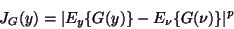(28)

where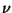is a standardized Gaussian random variable, y is assumed to be normalized to unit variance, and the exponent p=1,2 typically. The subscripts denote expectation with respect to y and. (The notation JG should not be confused with the notation for negentropy, J.)

Clearly, JG can be considered a generalization of (the modulus of) kurtosis. For G(y)=y4, JG becomes simply the modulus of kurtosis of y. Note that G must not be quadratic, because then JG would be trivially zero for all distributions. Thus, it seems plausible that JG in (28) could be a contrast function in the same way as kurtosis. The fact that JG is indeed a contrast function in a suitable sense (locally), is shown in [61,73]. In fact, for p=2, JG coincides with the approximation of negentropy given in (26).

In , the finite-sample statistical properties of the estimators based on optimizing such a general contrast function were analyzed. It was found that for a suitable choice of G, the statistical properties of the estimator (asymptotic variance and robustness) are considerably better than the properties of the cumulant-based estimators. The following choices of G were proposed: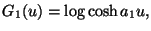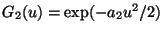(29)

where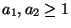are some suitable constants. In the lack of precise knowledge on the distributions of the independent components or on the outliers, these two functions seem to approximate reasonably well the optimal contrast function in most cases. Experimentally, it was found that especially the values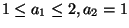for the constants give good approximations. One reason for this is that G1 above corresponds to the log-density of a super-gaussian distribution , and is therefore closely related to maximum likelihood estimation.Next: A unifying view on Up: One-unit contrast functions Previous: Higher-order cumulants
Aapo Hyvarinen
1999-04-23